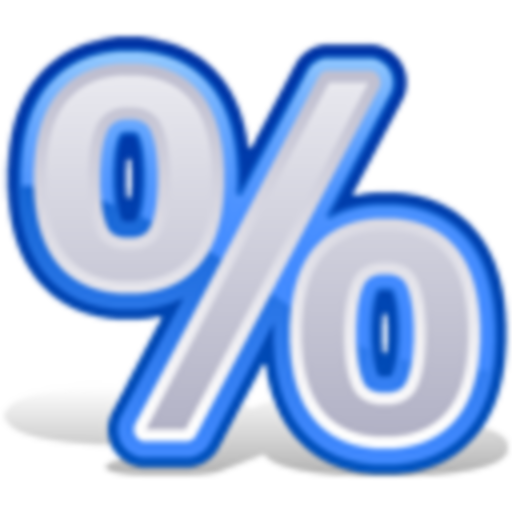# Percent Calculator

2.2 for Android
4.4 | 1,000,000+ Количество установок

WhichMan

## Описание для Percent Calculator

1. Calculate Percents & Percentages
2. Percent Discounts (sale price)
3. Percent Markups (increase by)
4. Percent Margin (selling price)
5. Calculate Tips.
6. Percentage Difference (Change)
7. Percentage (what % of) i.e. x is what percentage of y
Tip:
Enter any two values and the third is computed e.g. % of [x] = 115. X will be computed (20).
Customize background colors.

## Обновить Percent Calculator 2.2

e.g. What is [5%] of [29.99+14.99]

## Информация

• Категории:
Инструменты
• Последняя версия:
2.2
• Обновлено:
2013-10-03
• Размер файла:
257.6KB
• Требования:
Android 2.1 или более поздняя
• Обновлено:
WhichMan
• ID:
com.cityjams.calculators